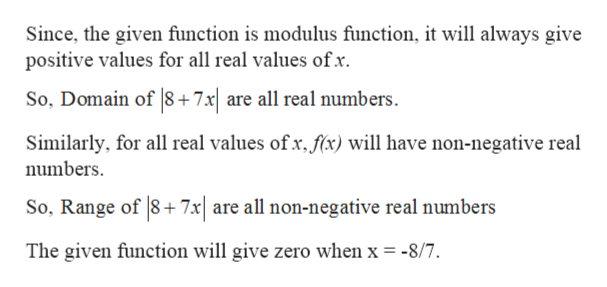# Find the domain and range of the function F(x)=|8+7x|.

Question
12 views

Find the domain and range of the function F(x)=|8+7x|.

check_circle

Step 1

Given: -

Step 2

To find: -

Step 3

Calculation...help_outlineImage TranscriptioncloseSince, the given function is modulus function, it will always give positive values for all real values ofx So, Domain of 8+ 7x| are all real numbers. Similarly, for all real values ofx, fx) will have non-negative real numbers So, Range of 8+7x| are all non-negative real numbers The given function will give zero when x = -8/7. fullscreen

### Want to see the full answer?

See Solution

#### Want to see this answer and more?

Solutions are written by subject experts who are available 24/7. Questions are typically answered within 1 hour.*

See Solution
*Response times may vary by subject and question.
Tagged in

### Functions